# AP Physics 2 Practice Test 14

### Test Information10 questions18 minutes

1.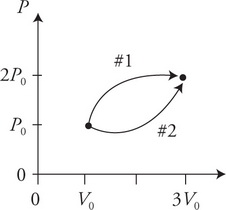A sample of gas can be taken from an initial pressure P0 and volume V0 to a final pressure and volume along either path 1 or 2 as shown in the figure. Your lab partner states: “Moving along either path won’t make any difference because both paths start and end at the same places. So, everything about the gases during both processes 1 and 2 will be the same.” Which of the following is a proper analysis of your lab partner’s statement?

2. A glass lens of focal length f = 80 cm in air is submerged in oil. How will submerging the lens in oil affect the focal length of the lens and why?

3.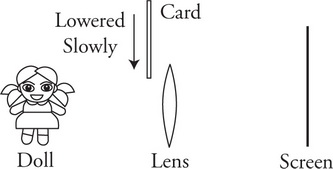A lens is placed between a doll and a white sheet of paper in such a way as to produce an image on the paper. On the side of the lens facing the doll, a dark card is slowly lowered to cover the lens as shown in the figure. Which of the following correctly explains what will happen to the image of the doll?

4.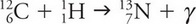Which of the following expressions correctly relates the masses of the constituent particles involved in the nuclear reaction shown?

5. In an experiment, monochromatic violet light shines on a photosensitive metal, which causes electrons to be ejected from the metal. Which of the following graphs best depicts the number of ejected electrons and the maximum energy of the ejected electrons versus the intensity of the violet light shining on the metal?

6.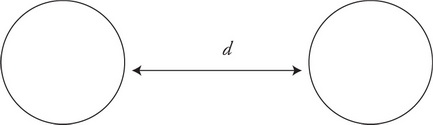Two identical insulating spheres of mass m are separated by a distance d as shown in the scale drawing. Both spheres carry a uniform charge distribution of Q. The magnitude of Q is much larger than the magnitude of m. What additional information is needed to write an algebraic expression for the net force on the spheres?

7.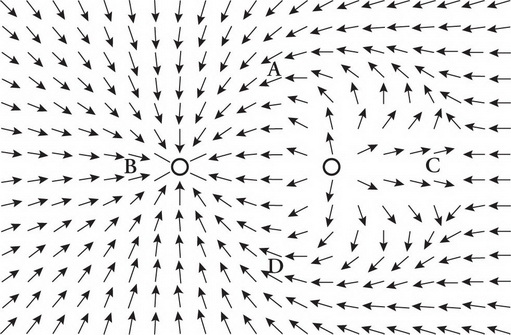The figure shows the electric field in a region surrounding two charges. The vectors in the diagram are not scaled to represent the strength of the electric field but show only the direction for the field at that point. Which two points have electric fields of the same magnitude?

8.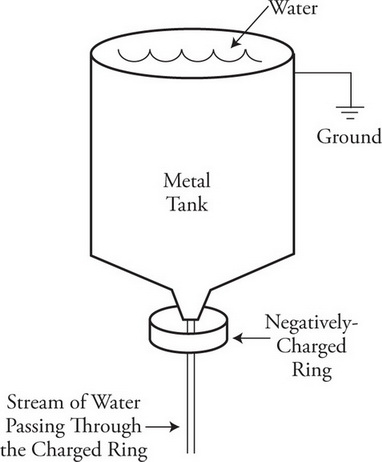A grounded metal tank containing water has a spout at the bottom through which water can flow out in a steady stream. A negatively charged ring is placed near the bottom of the tank such that the water flowing out of the tank passes through without touching the ring, as shown in the figure. Which of the following statements about the charge of the water flowing out of the tank is correct?

9.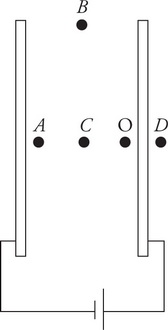Two parallel metal plates are connected to a battery as shown in the figure. A small negative charge is placed at location O and the force on the charge is measured. Compared to location O, how does the force on the charge change as it is moved to locations A, B, C, and D?

10.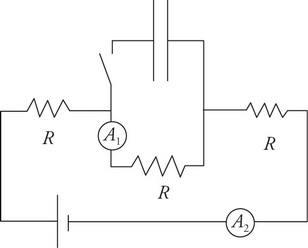The circuit shown in the figure consists of three identical resistors, two ammeters, a battery, a capacitor, and a switch. The capacitor is initially uncharged and the switch is open. Which of the following correctly compares the original open switch readings of the ammeters to their readings after the switch has been closed for a very long time?

A1     A2